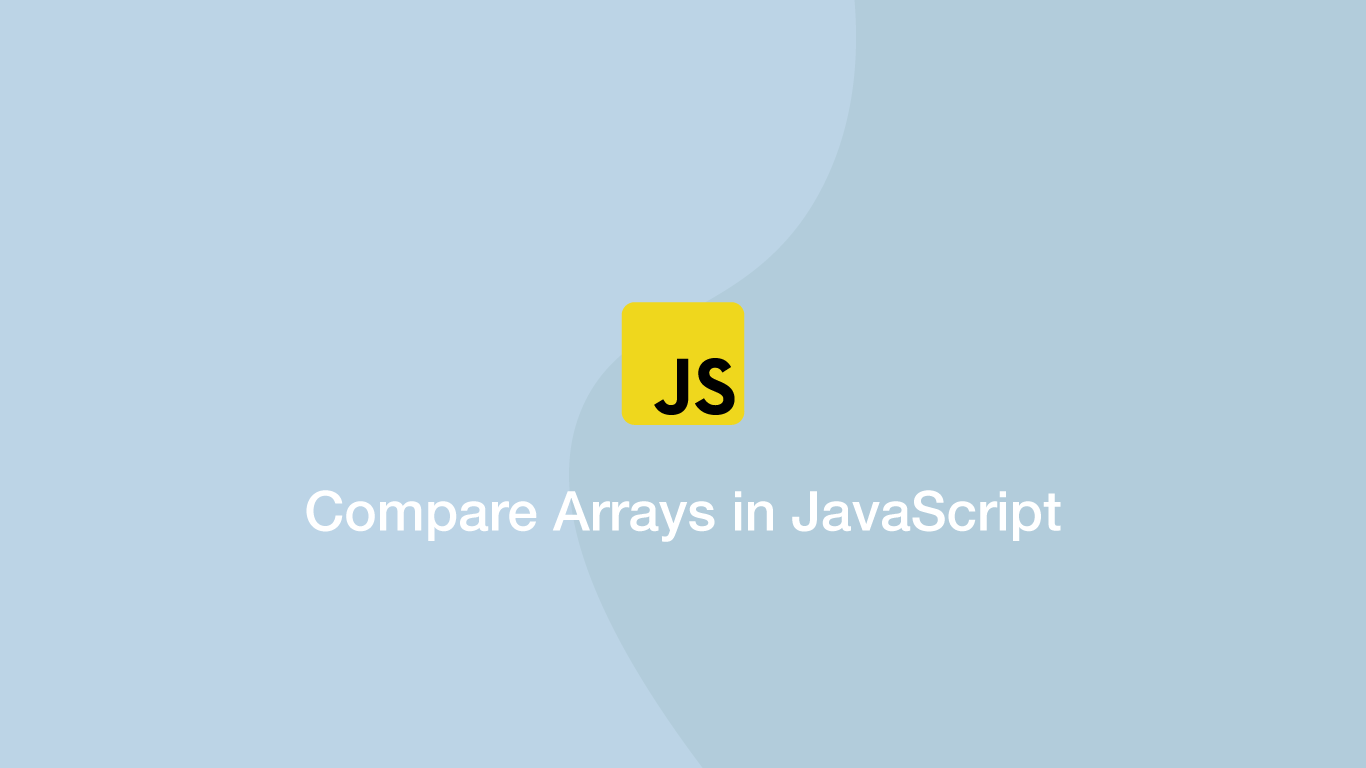# How to Compare Arrays in JavaScriptIn order to compare arrays in JavaScript, we first have to define what we want to compare – should the comparison be based on length, each value in the array or something else? In this tutorial, we will learn how to compare arrays in three different ways with examples.

## Compare Array Lengths

We can get the length of the array using the `.length` method, then it is just the case of comparing the two array lengths using strict equality.

``````fruit = ['apple', 'strawberry', 'peach', 'apricot', 'orange'];
fruit_2 = ['apple', 'strawberry', 'peach', 'apricot', 'orange'];

result = fruit.length == fruit_2.length;

console.log(result);
``````
``````true
``````

## Check if an Array has the same Reference

By reference I mean the do two variables reference the exact same array. This can be done using the strict equality comparison (`===`), which will only return `true` if the same array is referenced, not if the arrays are equal to each other.

``````fruit = ['apple', 'strawberry', 'peach', 'apricot', 'orange'];
fruit_2 = ['apple', 'strawberry', 'peach', 'apricot', 'orange'];

result = fruit === fruit_2;

console.log(result);
``````
``````false
``````
``````fruit = ['apple', 'strawberry', 'peach', 'apricot', 'orange'];
fruit_2 = fruit;

result = fruit === fruit_2;

console.log(result);
``````
``````true
``````

## Checking Each Value in Two Arrays is the Same

One way we could approach checking if an array has the same values as another is to loop through the first array and compare each of its values to the second one.

``````function arrayMatch(a, b) {

var result = true;

if (Array.isArray(a) && Array.isArray(b)) {
for (i in a) {
if (a[i] !== b[i]) {
result = false;
}
}
return result;
}
}

fruit = ['apple', 'strawberry', 'peach', 'apricot', 'orange'];
fruit_2 = ['apple', 'strawberry', 'peach', 'apricot', 'orange'];

console.log(arrayMatch(fruit, fruit_2));
``````

The above example will still return a `true` result so long as the first array is longer than the second. To change this behaviour to only compare arrays of the same length we could do something like this:

``````function arrayMatch(a, b) {

var result = true;

if (a.length !== b.length) {
return false;
}

if (Array.isArray(a) && Array.isArray(b)) {
for (i in a) {
if (a[i] !== b[i]) {
result = false;
}
}
return result;
}
}

fruit = ['apple', 'strawberry', 'peach', 'apricot', 'orange'];
fruit_2 = ['apple', 'strawberry', 'peach', 'apricot'];

console.log(arrayMatch(fruit, fruit_2));
``````
``````false
``````

## Conclusion

You now know three different ways of Comparing JavaScript arrays depending on what type of comparison you need to make. With the `for` loop approach, you have the ability to compare the values of more than two arrays or only check every n value matches its counterpart in another array using the remainder operator.

#### Related Tutorials### How to use the JavaScript reduce() Method

November 06, 2020### How to Compare Values in JavaScript

August 26, 2020### How to Create and Use Arrays in JavaScript

July 11, 2020### How to Merge Strings or Arrays in JavaScript Using the concat() Method

September 09, 2020### How to Compare Strings in Python

September 20, 2020July 16, 2020